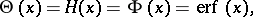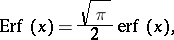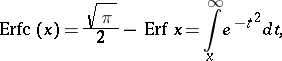# Probability integral

error integral

The function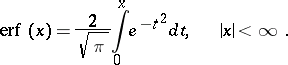In probability theory one mostly encounters not the probability integral, but the normal distribution function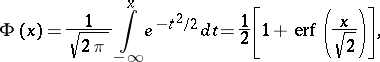which is the so-called Gaussian probability integral. For a random variablehaving the normal distribution with mathematical expectation 0 and variance, the probability that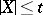is equal to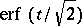. For real, the probability integral takes real values; in particular,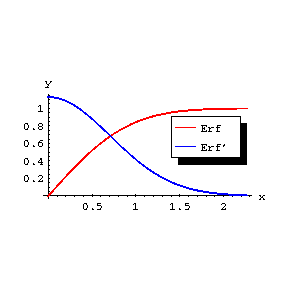Figure: p074920a

The graph of the probability integral and its derivatives are illustrated in the figure. Regarded as a function of the complex variable, the probability integralis an entire function of.

The asymptotic representation for large,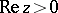, is given by: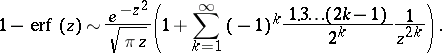In a neighbourhood ofthe probability integral can be represented by the series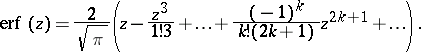The probability integral is related to the Fresnel integrals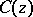and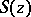by the formulas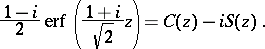The derivative of the probability integral is given by:The following notations are sometimes used: